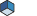# SoProjection Class Reference [Properties]Coordinate projection node More...

`#include <Inventor/nodes/SoProjection.h>`

Inheritance diagram for SoProjection:List of all members.

## Public Member Functions

virtual SoType getTypeId () const
SoProjection ()
void addProjection (SbString &projectionName, SbProjection *projection, int id)
void setProjection (SbProjection *projection)
void getCurrentProjectionInfos (SbString &name, int &id)
SbProjectiongetProjection () const

## Static Public Member Functions

static SoType getClassTypeId ()
static SbVec3fcheckProjection (SoState *state, SoNode *node, int32_t numCoords, const SbVec3f *coords)

## Public Attributes

SoSFBool isOn
SoSFMatrix matrix
SoSFInt32 projTypeId
SoMFFloat floatParameters
SoMFString parameters
SoMFString parametersIn

## Detailed DescriptionCoordinate projection node

This property node defines a coordinate projection that will be applied to all subsequent shapes in the scene graph. Coordinate transform nodes like SoTransform can only apply transforms that can be expressed as a matrix. A coordinate projection can be any algorithm that takes a coordinate and returns a different coordinate. This is particularly useful for geospatial coordinate systems (see SoGeoProjection), but is also useful for using spherical, cylindrical and other coordinate systems directly.

This node works together with projection classes derived from the SbProjection class. The projection classes implement the projection computations applied to 3D points. Applications can create new subclasses of SbProjection.

To use a specific projection, call setProjection() with an instance of the SbProjection class. Each instance of SoProjection can also store a list of projections, each one associated with a unique id. This is convenient for switching between different geospatial projections. Changing the projTypeId value activates the projection registered with the corresponding id.

The default projection is spherical coordinates (SbSphericalProjection).

The projection classes will use the string parameters and float parameters in the fields parameters and floatParameters respectively. See the projection class documentation for relevant parameters.

Normal vectors

• If no normal vectors are given for the geometry (and normal vectors are needed), Open Inventor will automatically compute normal vectors using the projected vertices, i.e. the coordinates after projection has been applied. This is usually the desired result.
• If normal vectors are specified for the geometry, they are not projected. Therefore normal vectors must be specified in post-projection (world) coordinates.

LIMITATIONS

• Transform nodes
The projection mechanism is not compatible with transform and manipulator nodes. Using nodes derived from SoTransformation, for example SoTransform or SoTabBoxManip will give unexpected results. To apply a transformation matrix to projected shapes, set the matrix field to the expected value. Shape vertices will be multiplied by the matrix before being projected.
• Memory
Because shape coordinates must be duplicated, memory consumption will increase when using projections.
• Picking
Projection is not applied when doing picking (SoRayPickAction). Results may be incorrect.

### FILE FORMAT/DEFAULT

Projection {  parameters "" matrix identity isOn true floatParameters 1 projTypeId 0
}

### ACTION BEHAVIOR

See related examples:

## Constructor & Destructor Documentation

 SoProjection::SoProjection ( )

Constructor.

## Member Function Documentation

 void SoProjection::addProjection ( SbString & projectionName, SbProjection * projection, int id )

Add a projection with the given name and id to the list.

The projection name is just for convenience. The projection id value should be greater than zero because the default spherical projection already uses this id. If multiple projections are assigned the same id, the first one added to the list will be used.

 static SbVec3f* SoProjection::checkProjection ( SoState * state, SoNode * node, int32_t numCoords, const SbVec3f * coords ) ` [static]`

Apply projection to given coordinates.

Store projected coordinates in current SoCoordinate3 element.

 static SoType SoProjection::getClassTypeId ( ) ` [static]`

Returns the type identifier for this class.

Reimplemented from SoNode.

Reimplemented in SoGeoProjection, and SoTransformProjection.

 void SoProjection::getCurrentProjectionInfos ( SbString & name, int & id )

Return current projection name and id.

 SbProjection* SoProjection::getProjection ( ) const` [inline]`

Return current projection.

 virtual SoType SoProjection::getTypeId ( ) const` [virtual]`

Returns the type identifier for this specific instance.

Reimplemented from SoNode.

Reimplemented in SoGeoProjection, and SoTransformProjection.

 void SoProjection::setProjection ( SbProjection * projection )

Set the current projection.

The specified projection is used regardless of whether it is in the list. In this case the value of the projTypeId field is not used.

## Member Data Documentation

Float parameters stored for projections.

If set to FALSE, projection is inactive.

Default is TRUE.

Matrix that can be applied before projecting a point.

Set to Identity by default

String parameters stored for projections.

String parameters stored for projections.

Current projection id.

Default is zero.

The documentation for this class was generated from the following file:

Open Inventor Toolkit reference manual, generated on 15 Mar 2023